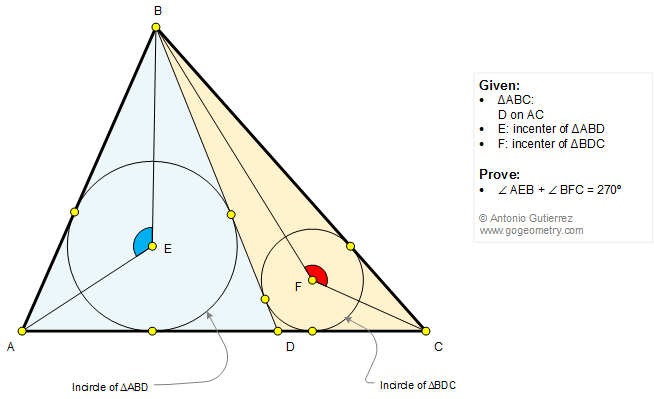# Geometry Problem 1326: Triangle, Cevian, Incenters, Sum of Angles, 270 Degrees. High School, College, Math Education

< PREVIOUS PROBLEM  |  NEXT PROBLEM >

 The figure below shows a triangle ABC with D on AC. E is the incenter of triangle ABD and F is the incenter of triangle BDC. Prove that angle AEB + angle BFC = 270 degrees.Home | Search | Geometry | Problems | All Problems | Open Problems | Visual Index | 10 Problems | Ten problems: 1321-1330 | Triangles | Incenter | Angles | Angle Bisector | Email | Solution / comment | by Antonio Gutierrez